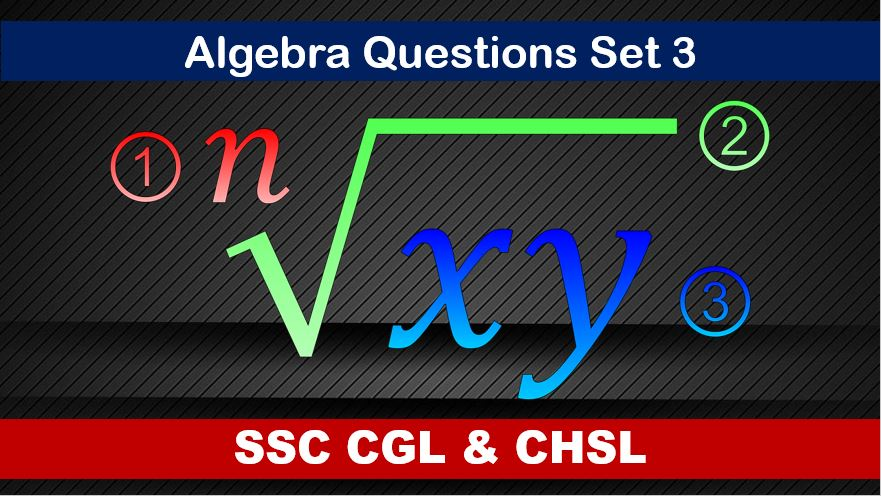Monday, September 27, 2021
Home > algebra > SSC CHSL Algebra Questions and Solutions Set 3

# SSC CHSL Algebra Questions and Solutions Set 3SSC CGL CHSL Algebra
Algebra Questions Sets
Set 1Set 2Set 3Set 4Set 5
Set 6

SSC CHSL Algebra Questions and Solutions Set 3 : Ques No 1

If x = 4ab/(a + b), where a ≠ b, then the value of (x + 2a) / (x – 2a) + (x + 2b) / (x – 2b) is

Options:

A. a

B. b

C. 2ab

D. 2

Solution:

SSC CHSL Algebra Questions and Solutions Set 3 : Ques No 2

If a + b + c = 0, then the value of 1/(a + b)(b + c) + 1/(a + c)(b + a) + 1/(c + a)(c + b) is

Options:

A. 1

B. 0

C. -1

D. -2

Solution:

SSC CHSL Algebra Questions and Solutions Set 3 : Ques No 3

If (a – 3)2 + (b – 4)2 + (c – 9)2 = 0, then the value of √(a + b + c) is

Options:

A. -4

B. 4

C. ±4

D. ±2

Solution:

SSC CHSL Algebra Questions and Solutions Set 3 : Ques No 4

If x + y = z, then the value of the expression x3 + y3 – z3 + 3xyz is

Options:

A. 0

B. 3xyz

C. -3xyz

D. z3

Solution:

SSC CHSL Algebra Questions and Solutions Set 3 : Ques No 5

If x + 1/x = 2, x ≠ 0, then the value of x2 + 1/x3 is

Options:

A. 1

B. 2

C. 3

D. 4

Solution:

SSC CHSL Algebra Questions and Solutions Set 3 : Ques No 6

If a2 = b + c, b2 = c + a and c2 = a + b, then the value of 1/(1 + a) + 1/(1 + b) + 1/(1 + c) is

Options:

A. abc

B. a2b2c2

C. 1

D. 0

Solution:

SSC CHSL Algebra Questions and Solutions Set 3 : Ques No 7

If a + 1/a + 2 = 0, then the value of (a37 – 1/a100) is

Options:

A. 0

B. -2

C. 1

D. 2

Solution:

SSC CHSL Algebra Questions and Solutions Set 3 : Ques No 8

If x + y + z = 6, then the value of (x – 1)3 + (y – 2)3 + (z – 3)3 is

Options:

A. (x – 1)(z – 3)

B. (y – 2)(z – 3)

C. 3(x – 1)(y – 2)(z – 3)

D. 4(x2 + y2 + z2)

Solution:

SSC CHSL Algebra Questions and Solutions Set 3 : Ques No 9

If x = √3 + √2, then the value of (x + 1/x) is

Options:

A. 2√2

B. 2√3

C. 2

D. 3

Solution:

SSC CHSL Algebra Questions and Solutions Set 3 : Ques No 10

If x + 1/x = 3, then the value of (x3 + 1/x) / (x2 – x + 1) is

Options:

A. 3/2

B. 5/2

C. 7/2

D. 11/2

Solution:

SSC CHSL Algebra Questions and Solutions Set 3 : Ques No 11

The ages of the two persons differ by 20 years. If 5 year ago, the older one be 5 times as old as the younger one, then their present ages, in year are

Options:

A. 25, 5

B. 30, 10

C. 35, 15

D. 50, 30

SSC CHSL Algebra Questions and Solutions Set 3 : Ques No 12

A student was asked to divide a number by 6 and add 12 to the quotient. He, however first added 12 to the number and then divided it by 6, getting 112 as the answer. The correct answer is

Options:

A. 114

B. 118

C. 122

D. 124

SSC CHSL Algebra Questions and Solutions Set 3 : Ques No 13

A father with 8 children takes 3 children at a time to the zoological garden, as often as he can without taking the same 3 children together more than once. Then

Options:

A. number of times he will go to zoological garden is 56.

B. number of times each child will go to the zoological garden is 21.

C. number of times a particular child will not go to the zoological garden is 35

D. All of the above.